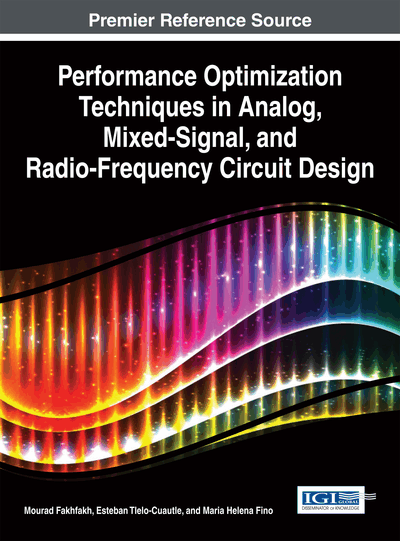# Stability of Optimization Trajectories for Designing Analog Circuits

Alexander Zemliak (Autonomous University of Puebla, Mexico & National Technical University of Ukraine, Ukraine)
Copyright: © 2015 |Pages: 31
DOI: 10.4018/978-1-4666-6627-6.ch004
OnDemand PDF Download:
Available
\$37.50
No Current Special Offers

## Abstract

In this chapter, the problem of computer time reduction for optimization of large electronic system is discussed. It is one of the essential problems of high-quality improvement of design, and it is defined by means of the generalized methodology for analog network optimization on the basis of the control theory formulation. It is shown that the main conception of this methodology is based on a special control vector which operates process of optimization and gives a solution of optimization problem during the minimal computing time. The problem of creation of a vector of optimal control is solved on the basis of direct Lyapunov method. Lyapunov function of optimization process is proposed to analyze a stability of trajectories of optimization. This function gives the opportunity of the analysis of stability of various strategies and is used as the basis for search of the optimal algorithm of designing.
Chapter Preview
Top

## Introduction

The problem of the computer time reduction of designing of large system is one of the essential problems of the total quality design improvement. The design problem involves determining of all the specifications that needed to obtain for the circuit. The traditional process of analog circuit optimization includes a definition of topology of circuit, the starting point in a space of circuit’s variables and methods for circuit analysis and parametric optimization. This process is needed to define numerical values for the components of the circuit. The process of parameters adjusting can be based on optimization procedure. Mathematically this process is defined as a minimization of special objective function that includes necessary features of the projected circuit. It means that any strategy of circuit designing includes two main blocks: the analysis of the mathematical model of circuit and the optimization procedure that achieves the point of minimum of objective function. The minimal value of this function can guarantee obtaining the necessary specifications of the circuit.

There are some powerful methods that reduce the necessary time for the circuit analysis. Because a matrix of a large-scale circuit is a very sparse, the special sparse matrix techniques are used successfully for this purpose (Duff and Reid, 1979; Osterby & Zlatev, 1983; George, 1984). Other approach to reduce the amount of computational required for the linear and nonlinear equations are based on the decomposition techniques. The partitioning of a circuit matrix into bordered-block diagonal form can be done by branches tearing as in (Wu, 1976), or by nodes tearing as in (Sangiovanni-Vincentelli, Chen & Chua, 1977) and jointly with direct solution algorithms gives the solution of the problem. The extension of the direct solution methods can be obtained by hierarchical decomposition and macromodel representation (Rabat, Ruehli, Mahoney & Coleman, 1985). Other approach for achieving decomposition at the nonlinear level consists on a special iteration techniques and they have been realized for example in (Ruehli, Sangiovanni-Vincentelli & Rabbat, 1982), (George, 1984) for the iterated timing analysis and circuit simulation.

The methods of analysis of complex systems are improved constantly and some alternative methods like symbolic methods (Gielen et al. 1990; Yu and Sechen, 1996) and homotopy methods (Liao, 2004; Tadeusiewicz and Kuczynski, 2013) were applied successfully for the analysis of nonlinear circuits.

Optimizing methods also have a very strong impact on the general properties of process of circuit design. Different techniques for analog circuit optimization can be classified in two groups: deterministic optimization algorithms and stochastic search algorithms. Deterministic methods of optimization were developed during some decades for different applied problems. Some well-known mathematical methods are applied successfully for analog circuit optimization. These are the methods of the first and second order (like gradient and Newton methods) and methods of the order 1.5 like Davidon-Fletcher-Powell method. The achievements in developing of deterministic mathematical methods of optimization (Fletcher, 1980; Gill et al., 1981) produce some perspective directions for the unconstrained and constrained optimization. Practical methods are developed for VLSI circuit designing, timing and area optimization (Brayton et al., 1981; Ruehli, 1987).

## Complete Chapter List

Search this Book:
Reset